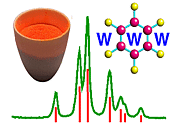X-ray Filters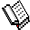Course Material Index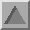Section Index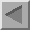Previous Page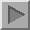Next Page

X-ray Filters

Function

The previous pages showed that the spectrum from a sealed X-ray tube is composed of several X-ray lines. Laboratory powder diffraction requires an X-ray source that is essentially monochromatic and so the Kβ line in the X-ray spectrum needs to be removed. Metal foil filters are one way of achieving this.

The photograph shows the typical metals used to filter X-rays produced by a sealed X-ray tube, i.e. Ni, Fe, Mn, V, or Zr. Filters preferentially reduce the intensity of the Kβ line in the X-ray spectrum compared to Kα as explained below. Note that absorption filters cannot be used to remove the unwanted Kα2 component from Kα radiation.

Filters exploit the X-ray absorption edge of the particular element. At wavelengths longer than the absorption edge (i.e. just above the edge), the absorption of the X-rays is considerably less than for wavelengths shorter than the absorption edge (i.e. just below the edge) as shown below for nickel metal: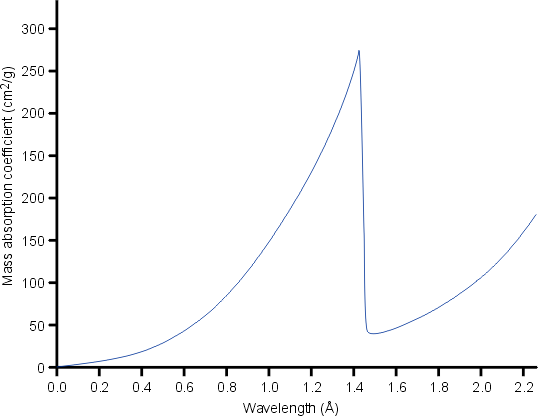The absorption edge of nickel metal at 1.488 Å lies between the Kα (λ = 1.542 Å) and Kβ (λ = 1.392 Å) X-ray spectral lines of copper. Hence nickel foil of an appropriate thickness can be used to reduce the intensity of the Cu Kβ X-rays as shown below: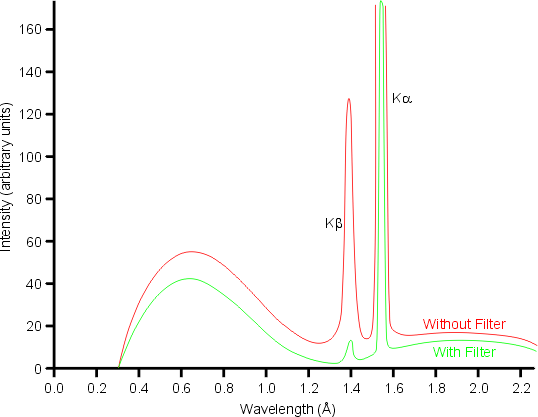Note that the filter also removes much of the high energy background radiation.

The choice of filter material depends upon the choice of anode material in the X-ray tube as shown in the following table:

 Anode Filter Cu Co Fe Cr Mo Ni Fe Mn V Zr

From the table it can be seen that the ideal choice of material for an X-ray filter is a metal whose atomic number, Z, is one less than that of the anode target metal for first row transition metals (or two less for second row transition metals).

I(λ) / Io(λ) = exp{− (μ / ρ)λ ρx}
where (μ / ρ) is the mass absorption coefficient at the wavelength λ, ρ is the density of the material, which for nickel metal is 8.92 g/cm3, and I(λ) and Io(λ) are the transmitted and incident X-ray intensities, respectively. The mass absorption coefficients of nickel for Cu Kα and Cu Kβ are 49.2 and 286 cm2/g, respectively. The table below shows the percentage transmission for various thicknesses of nickel foil:
Thickness (cm)   I / Io (%) for Cu Kα   I / Io (%) for Cu Kβ   Reduction Ratio
0.0010  64.5  7.8 8
0.0015  51.8  2.2 24
0.0020  41.6  0.6 68
0.0025  33.4  0.2 197

It can be seen from the table that the optimum thickness has to be a compromise between reducing the intensity of the unwanted Cu Kβ and reducing the intensity of the desired Cu Kα. Most commercial systems employing a nickel filter with a copper anode target will choose the thickness of the foil so as to give a reduction ratio in the range 25:1 to 50:1, i.e. foils between 15 and 20 µm thick. From the table, it can be seen that this range of foil thickness will diminish the desired radiation by approximately a factor of 2.Course Material IndexSection IndexPrevious PageNext Page
 © Copyright 1997-2006.  Birkbeck College, University of London. Author(s): Jeremy Karl Cockcroft